## Excel FunctionsThe sidebar (or click the hamburger icon if you are on mobile) shows a list of Excel functions based on category. Press CTRL + F in your web browser to quickly search the sidebar for the function you are looking for.

If you rather prefer an alphabetically sorted list then this link takes you to Excel functions sorted from A to Z.

### How to enter a function in Excel

You are now shown the arguments for the chosen function.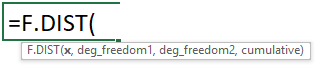Enter the values for each argument, some arguments display a list of options if the function requires you to enter one of many predetermined parameters, see image below.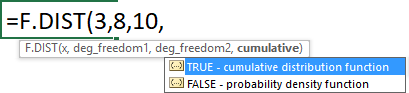Use the arrow keys to select an argument and press TAB key to choose the selected argument. Type a closing parentheses when all arguments have been entered.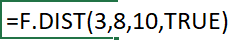Lastly press ENTER or CTRL + SHIFT + ENTER if the function requires you to.

### How do I insert a function?1. Select a cell.
2. Click the "fx" button next to the formula bar, a dialog box appears.
3. The dialog box allows you to search for a function. You can also browse functions based on category or recently used functions.
4. The dialog box also specifies the arguments of the selected function which is great if you are not familiar with the selected function.
5. At the very bottom of the dialog box is a link that takes you to Microsofts support page based on the function you selected.
6. Click the OK button.

This takes you to another dialog box that guides you through each argument in the selected function.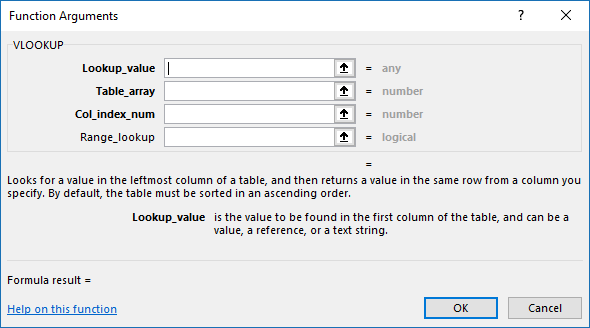The dialog box describes each argument and lets you select cell(s) in your worksheet in order to complete the function.

Click the OK button when all arguments are specified.

### How many arguments in a function can I use?

It depends on the function, some lets you use up to 255 arguments and others have none. For example, the SUM function allows you to have up to 255 arguments in one function where as the TODAY function has no arguments.

An argument may contain a cell reference to a single cell or a cell reference to multiple cells, constants, arrays or structured references.

### What are the most used functions of Excel?

Excel provides you functions to perform mathematical, statistical and financial calculations.

The most used functions are probably:

### What are the most useful functions?

It depends who ask, users may have different goals. In general, if you have a task that is tedious and time-consuming there is a great chance there is a feature or function that can help you out.

Start by doing a internet search on what you want to accomplish in Excel, most likely there are others that have had the same problem/question.

Many consider the VLOOKUP function or INDEX - MATCH functions to be immensely powerful. They allow you to do lookups and return another value on the same row as the matching value.

In fact, there are som many functions that most problems you encounter can be solved. Excel lets you also, in most cases, combine several functions in order to build a formula that is exactly what you are looking for.

### How many functions are there in Excel?

It depends on your Excel version, older versions have fewer functions. There are at least 467 different functions in the latest version.

### Are the functions categorized?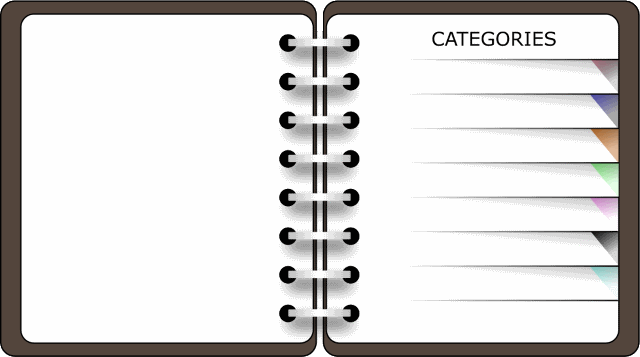Yes, there are at least 12 different categories.

### What are the latest Excel functions?

The following six functions are the latest in the Office 365 subscription:

### What functions are outdated?

Some functions have been replaced by newer better functions, you can find them in the Compatibility category. They are still working, however, they may be removed in future updates. I recommend that you replace the outdated functions with their newer equivalents.

### What is an Excel formula?

A formula calculates a value based on the function or functions used. It always begins with a equal sign so Excel can distinguish it from a text or numerical value.

### What is an array formula?

In general, an array formula calculates multiple values simultaneously and sometimes but not always return multiple values.

To enter an array formula, type the formula in a cell then press and hold CTRL + SHIFT simultaneously, now press Enter once. Release all keys.

The formula bar now shows the formula with a beginning and ending curly bracket telling you that you entered the formula successfully. Don't enter the curly brackets yourself.

This article demonstrates this in greater detail: How to enter an array formula

### What functions are you required to enter as an array formula?

In general, functions that return more than one value requires you to enter them as an array formula. You must enter the following functions as an array formula in order to make it work properly.

You can also use regular functions in an array formula and when you start doing that you are beginning to discover all the really powerful stuff that is possible with Excel.

### What is a volatile function?

A volatile function is a function that is recalculated every time the worksheet is calculated. What this means is that it may slow down your workbook considerably.

For example, the TODAY function returns the date each time the worksheet is recalculated to make sure the current day is displayed. This is not cpu intensive if entered in a single cell, however, hundreds or thousands of cells then you may notice a difference.

The following functions are volatile:

### How do I troubleshoot a function/formula?Excel has a built-in feature that allows you to see each step in the formula calculation, this makes it easier to see which function is giving you trouble.

1. Select the cell containing the formula you want to troubleshoot.
2. Go to tab "Formulas" on the ribbon.
3. Click "Evaluate Formula" button, a dialog box appears.
4. Click the "Evaluate" button to see each calculation step.

You can also right-click on the cell to see a context menu. This menu allows you to investigate the error further, you can see a descriptive text of the error message.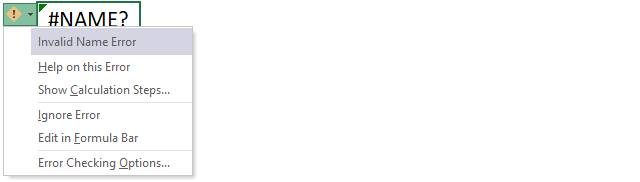The second line allows you to see the support page of this error, the third line opens the "Evaluate formula" tool that I demonstrated above.

### What is a cell reference?

A cell reference is an address to a specific cell in your workbook. Each column is labeled from A to XFD and rows are numbered from 1 to 1048576, a cell reference contains the column letter and the row number like coordinates.

Example, here is a cell reference: Sheet1!G34. Sheet1 is the name of the worksheet the cell is located on. G is the column which is the seventh column from the left, 34 is the row number.